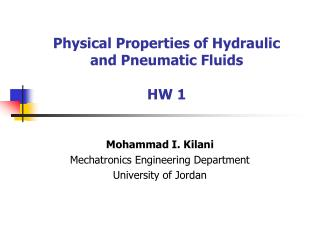DownloadDownload PresentationPhysical Properties of Hydraulic and Pneumatic Fluids HW 1

Physical Properties of Hydraulic and Pneumatic Fluids HW 1

Télécharger la présentationPhysical Properties of Hydraulic and Pneumatic Fluids HW 1

- - - - - - - - - - - - - - - - - - - - - - - - - - - E N D - - - - - - - - - - - - - - - - - - - - - - - - - - -
Presentation Transcript

1. Physical Properties of Hydraulic and Pneumatic FluidsHW 1 Mohammad I. Kilani Mechatronics Engineering Department University of Jordan

2. F 2 kN L HW 1 Output speed of a Hydraulic Jack • The hydraulic jack has an input area of 10 mm2 and an output area of 40 mm2, and is used to move the output piston against a constant 2kN load with different output speed values. The input cylinder is connected to the output cylinder with a 4 mm2 pipe of length L. The density of the hydraulic fluid used in the jack is 900 kg/m3 and its viscosity is 0.3 kg.m/s2. • Plot the force F needed on the input piston as a function of the steady output piston speed. Generate three different curves on the same plot corresponding to (i) L = 5 m, (ii) L = 10 m and (iii) L = 20 m. Identify all the critical inflection points on the plot. • For F = 1 kN and L = 20 m, derive an expression for the transient output speed, v, as a function of time, t, and find the time needed for the piston to reach 95% of the steady speed. Plot v(t) using appropriate scale.

3. F 2 kN L HW 1 Output speed of a Hydraulic Jack • Under steady operating conditions, the weight of the 2kN load is balanced by the force of the oil pressure • For laminar flow in the connecting pips, Re = 2300 • The hydraulic jack has an input area of 10 mm2 and an output area of 40 mm2, and is used to move the output piston against a constant 2kN load with different output speed values. The input cylinder is connected to the output cylinder with a 4 mm2 pipe of length L. The density of the hydraulic fluid used in the jack is 900 kg/m3 and its viscosity is 1.1 x 10-7 kg.m/s2. • Plot the force F needed on the input piston as a function of the steady output piston speed. Generate three different curves on the same plot corresponding to (i) L = 5 m, (ii) L = 10 m and (iii) L = 20 m. Identify all the critical inflection points on the plot.